Courses

# Test: Data Handling - 3

## 20 Questions MCQ Test Mathematics (Maths) for Class 4 | Test: Data Handling - 3

Description
This mock test of Test: Data Handling - 3 for Class 4 helps you for every Class 4 entrance exam. This contains 20 Multiple Choice Questions for Class 4 Test: Data Handling - 3 (mcq) to study with solutions a complete question bank. The solved questions answers in this Test: Data Handling - 3 quiz give you a good mix of easy questions and tough questions. Class 4 students definitely take this Test: Data Handling - 3 exercise for a better result in the exam. You can find other Test: Data Handling - 3 extra questions, long questions & short questions for Class 4 on EduRev as well by searching above.
QUESTION: 1

Solution:
QUESTION: 2

Solution:
QUESTION: 3

### Questions are based on the following pictograph. In the following bar graph run scored by Rahul Dravid in five matches in a tournament has been shown: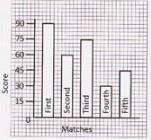Q. How many runs Rahul Dravid scored in the tournament?

Solution:
QUESTION: 4

Questions are based on the following pictograph.
In the following bar graph run scored by Rahul Dravid in five matches in a tournament has been shown: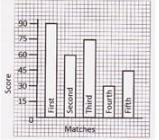Q. What was the highest score of Rahul Dravid in the tournament?

Solution:
QUESTION: 5

Questions are based on the following pictograph.
In the following bar graph run scored by Rahul Dravid in five matches in a tournament has been shown: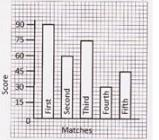Q. What is the lowest score of Rahul Dravid in the tournament?

Solution:
QUESTION: 6

Direction for questions: Class-4 did a survey of their favourite types of story book.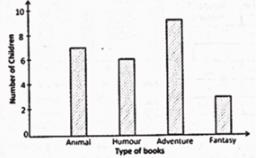Q. How many more children chose adventure books than fantasy books?

Solution:
QUESTION: 7

Direction for questions: Class-4 did a survey of their favourite types of story book.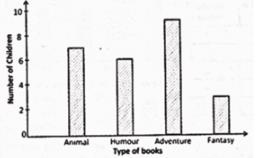Q. How many children chose humour books?

Solution:
QUESTION: 8

Direction for questions: Class-4 did a survey of their favourite types of story book.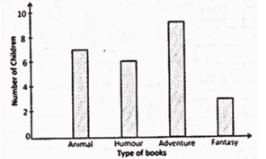Q. Which type of book is preferred by maximum children?

Solution:
QUESTION: 9

Direction for questions: Class-4 did a survey of their favourite types of story book.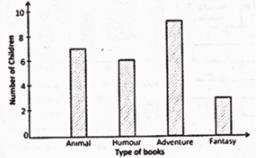Q. How many more children chose animal books than humour books?

Solution:
QUESTION: 10

Direction for questions: Class-4 did a survey of their favourite types of story book.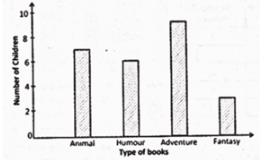Q. Which type of book is preferred by minimum children?

Solution:
QUESTION: 11

Direction for questions: 60 children visit the zoo. They each vote for their favourite animal.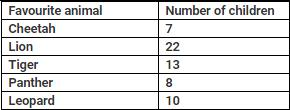Q. Maximum number of children voted for

Solution:
QUESTION: 12

Direction for questions: 60 children visit the zoo. They each vote for their favourite animal.Q. How many children voted for Leopard?

Solution:
QUESTION: 13

Direction for questions: 60 children visit the zoo. They each vote for their favourite animal.Q. How many more children voted for Tiger than Panther?

Solution:
QUESTION: 14

Direction for questions: 60 children visit the zoo. They each vote for their favourite animal.Q. Minimum number of children voted for

Solution:
QUESTION: 15

Direction for questions: 60 children visit the zoo. They each vote for their favourite animal.Q. 13 children voted for

Solution:
QUESTION: 16

DIRECTION: The given pictograph tells us about the kind of books Mohan has read. Study the pictograph carefully and answer the following questions'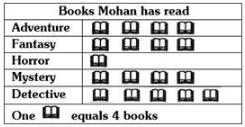Q. How many books did Mohan read in all?

Solution:

Total number of books Mohan read = 4 × 18 = 72

QUESTION: 17

DIRECTION: The given pictograph tells us about the kind of books Mohan has read. Study the pictograph carefully and answer the following questions'Q. How many detective books did he read?

Solution:

Number of detective books he read = 4 × 5 = 20.

QUESTION: 18

DIRECTION: The given pictograph tells us about the kind of books Mohan has read. Study the pictograph carefully and answer the following questions'Q. How many Fantasy books did he read?

Solution:

Number of Fantasy books he read = 4 × 4 = 16

QUESTION: 19

DIRECTION: The following table shows the number of bulbs sold by 4 factories over a period of 4 months.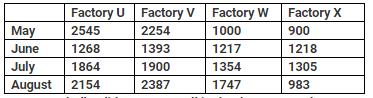Q. How many bulbs did Factory U sell in the these 4 months?

Solution:

Number of bulbs Factory U sell in given 4 months = 2545 + 1268 + 1864 + 2154 = 7831

QUESTION: 20

DIRECTION: The following table shows the number of bulbs sold by 4 factories over a period of 4 months.Q. How many bulbs did Factory V sell more than Factory W in May and June?

Solution:

Number of bulbs sold by Factory V in May and June = 2254+1393=3647
Number of bulbs sold by Factory W in May and June =1000+1217=2217
∴ Required number of bulbs = 3647−2217= 1430